﻿ Students’ Use of Intuitive Reasoning to Decide on the Validity of Mathematical Statements

### Students’ Use of Intuitive Reasoning to Decide on the Validity of Mathematical Statements

Chih-Hsien Huang

American Journal of Educational Research

## Students’ Use of Intuitive Reasoning to Decide on the Validity of Mathematical Statements

Chih-Hsien HuangMing Chi University of Technology

### Abstract

This study explored students’ approaches to mathematical statements with unknown truth values. Task-based interviews utilizing the think-aloud method revealed students’ reasoning processes in depth. The students in this study used three distinct types of intuitive reasoning to decide the truth value of mathematical statements. The results of this study indicate that in each intuitive response there seems to be an underlying logical schema, or a mental model intuitively accepted by the students, which interacts with the specific restrictions of the presented mathematical statement.

• Chih-Hsien Huang. Students’ Use of Intuitive Reasoning to Decide on the Validity of Mathematical Statements. American Journal of Educational Research. Vol. 4, No. 14, 2016, pp 1025-1029. http://pubs.sciepub.com/education/4/14/7
• Huang, Chih-Hsien. "Students’ Use of Intuitive Reasoning to Decide on the Validity of Mathematical Statements." American Journal of Educational Research 4.14 (2016): 1025-1029.
• Huang, C. (2016). Students’ Use of Intuitive Reasoning to Decide on the Validity of Mathematical Statements. American Journal of Educational Research, 4(14), 1025-1029.
• Huang, Chih-Hsien. "Students’ Use of Intuitive Reasoning to Decide on the Validity of Mathematical Statements." American Journal of Educational Research 4, no. 14 (2016): 1025-1029.

 Import into BibTeX Import into EndNote Import into RefMan Import into RefWorks

1
Prev Next

### 1. Introduction

Many educators believe that students' desire for proof will be stimulated by opportunities to explore the truth value of mathematical statements. A main challenge in teaching argumentation and proof is to motivate students to examine whether and why statements are true or false . Unfortunately, in the standard process of mathematics teaching, students are seldom required to construct proofs of unknown statements or to determine the truth value of mathematical statements [4, 5]. Because of the emphasis on syntactic reasoning and prove this statements in the undergraduate curriculum , little is known about how engineering students approach mathematical statements with unknown truth values.

Intuition is particularly important for determining the truth value of a mathematical statement, because in the absence of proof, it provides possibilities that students can then test [2, 9]. This study attempts to explore the use of intuitive reasoning and what types of systematic errors may inhibit success in the proving process during the processes of deciding on the truth value of mathematical statements by engineering students in an interview setting.

### 2. Theoretical Framework

The intuition proposed by Fischbein is a representation, an explanation or an interpretation directly accepted by us as something natural, self-evident, intrinsically meaningful, like a simple, given fact . Intuition takes into consideration the target of reasoning in prior knowledge, experience, conviction, task characteristics, and the creation of task representation . Furthermore, "intuition is able to organize information, to synthesize previously acquired experiences . . . to guess, by extrapolation, beyond the facts at hand" . Organizing information intuitively provides a preliminary understanding of mathematical tasks which can provide a starting point and suggest a direction can be followed [2, 7, 8]. Intuitive representation in mathematics may be either a visual image or a perceptual representation of a concept or object .

Fischbein pointed out intuition is neither a source nor a method; it is a form of cognition . Unlike analytical thinking, it is a holistic leap of cognition. In his view, experience plays a crucial role in developing intuition. On the basis of stable and consistent experience, a thinker may learn to rely upon intuition, and it is quite autonomous in special circumstances. It may also impact individual judgment. Fischbein's classification is designed to clarify the complicated areas of intuitive cognition into two main types . The first type is classified according to the roles played by intuition (affirmatory, conjectural, anticipatory, and conclusive), and the second type by the origin of intuition (primary and secondary). Fischbein distinguished between affirmatory intuitions, which he described as direct and self-evident cognition without the need for checking or proving, and anticipatory intuitions, a sense of intrinsic conviction of one's ideas without any extrinsic encouragement .

Intuition is based on mental representations of tasks constructed from the clues given in a task and from the information retrieved from memory . This production of representations makes intuition significant in decision-making. Due to the inconsistency and incorrectness of previous learning experiences, the intuitive representation of individuals may not be able to authentically present the situation at hand. The reliability of intuition often depends on how intuition develops through related experiences [2, 6]. Many intuitive errors can be categorized as accessibility errors . Accessibility is the ease with which certain knowledge is evoked or certain task features are perceived and is a crucial component of intuitive reasoning and decision-making. There are two main types of accessibility errors, namely (1) attribute substitution, and (2) knowledge and task feature relevance.

### 3. Method

This research has interpretive approaches. Case study is used as a research strategy to make an in-depth examination of students’ intuitive reasoning in this study . The 23 first-year engineering students who participated in this study were enrolled at a university of technology and had learned the concepts of derivative and integration. This study explores the results of this process among engineering students rather than mathematicians, a choice more likely to produce values in teaching and "suggest learning trajectories that might be applicable for many other students as well" . The mathematical task in this paper included two wrong statements regarding the concepts of differentiation and integration. For students, they were neither completely routine problems nor completely non-routine ones. The tasks refer to general objects and their properties and should be amenable to intuitive reaction. Participants completed the tasks in which they were asked to determine the truth value of the given mathematical statements and prove or disprove the statement accordingly.

Statement 1: If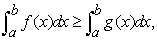then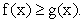True or false? Justify your answer.

Statement 2: If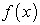and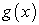are both differentiable and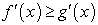,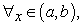then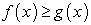,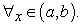True or false? Justify your answer.

The students were asked to determine the accuracy of the mathematical statements and justify their answers. Data were gathered concerning the examples (counterexamples) that were produced by the participants. Each student worked individually on each problem.

The data generated from (a) transcripts from the participants’ task-based interviews using the think-aloud method, and (b) participants’ written work on the tasks in the interviews, and (c) my field notes from the interviews were categorized and coded . As the process evolved, continuous comparisons were made between each category and the emerging new categories. Additionally, students’ decision-making and construction processes were analyzed to determine students’ decision-making and the connections between these processes. I will classify reasoning as intuitive if the student (a) stated that it was an intuition, instinct, gut feeling, or first thought; (b) used similarity to make an assessment of the task; or (c) easily made immediate transformations of signs within and between semiotic systems.

The analysis process yielded a categorization of the data around two major themes: (a) characterizing the subjects' intuitive reasoning as reflected in their task performance; and (b) characterizing the subjects' visual reasoning as reflected in their task performance. Each of these two themes was subcategorized.

Theme (a), subjects' intuitive reasoning, included the following:

(a) 1. Logic-based intuitive reasoning.

(a) 2. Property-based intuitive reasoning.

(a) 3. Similarity-based intuitive reasoning

Theme (b), subjects' intuitive representations, included the following:

(b) 1. The graphs of function f and g are the two non-intersect curves located above the x axis.

(b) 2. The graphs of function f and g are the two non-intersect curves located beneath the x axis.

(b) 3. The graphs of function f and g intersect

(b) 4. The graphs of function f and g are two intersecting straight lines with different slopes.

### 4. Empirical Data and Analysis

The students used three distinct types of intuitive reasoning to decide the truth value of mathematical statements.

4.1. Logic-based Intuitive Reasoning

Logic-based intuition was the first type of intuitive reasoning used by students. It occurred only when determining the truth value of Statement 1. Three students made a logical mistake when determining the truth value of Statement 1. They intuitively believed that the mathematical statement and its converse are equivalent. For instance, the converse that S4 made in judging Statement 1 was correct, and hence Statement 1 is correct.

S4: A greater integral correspond to a larger function. For example, given f = x2+1 and g = x2, f is larger than g. The integral of f from 0 to 1 is 4/3, which is also greater than the integral of g, 1/3, over the same interval.

I: Did you find out f(x) and g(x) in the first place and that f(x) is greater than g(x), and then figured out that the integral of f(x) is greater than that of g(x).

S4: That's right! The greater the function is, the greater the integral will be.

I: But Statement 1 says the integral is relatively greater, does this mean that the function is greater, too? The example that you provided just now indicates that the greater the function is, the greater the integral will be.

S4: Indeed. They work in the same way. If the function becomes greater, the integral will be greater as well, and vice versa.

According to Fischbein, we can confirm that the equivalence of a statement and its converse (error) is a kind of intuition. Is it true that these three students did not possess formal logical schemas? Apparently this was not the case.

I: If f(x) is differentiable, will f(x) be continuous?

S4: Yes, f(x) is differentiable, so f(x) is continuous.

I: If f(x) is continuous, will f(x) be differentiable?

S4: If f(x) is continuous, I am not sure whether f(x) is differentiable. It is certain that if f(x) is discontinuous, then it could not be differentiated.

Apparently, a sufficient condition for determining the truth value of mathematics does not rely on whether the logical rules are well understood, and the student did not correctly apply logical rules to other scenarios. This supports the argument by Fischbein, that intuitions are not absolute, they depend on the context . We can interpret these phenomena by describing two convictions that may coexist : The first one is intuitive conviction, which means that a statement and its converse are equivalent; the second one is non-intuitive conviction, which means a statement and its contrapositive are equivalent. These three students like S4 could write out the statement that contrapositive is equivalence when elaborating on the relationship between differentiation and continuity. However, when determining the truth value of mathematical statement 1, more of their intuitive conviction came into play; they also confirmed that a statement and its converse are equivalent. In Fischbein's words, the first one was an intuitive intrinsic type of conviction, and the second one was a formal extrinsic type of conviction. In our view, the latter seems to have no impact on the former, which continued to be an obstacle.

4.2. Property-based Intuitive Reasoning

The second type of intuitive reasoning students used was property-based intuition. Students in this subgroup drew quick conclusions about the truth value of mathematical statements by using diagrams to represent "prototypical" examples of such mathematical statements. When confronting Statement 1, the property that students immediately thought of was area, and subsequently they directly used region areas surrounded by functional graphs to decide on the truth value of mathematical statements. Generally speaking, the students produced two different types of intuitive representation according to their intuitive representations. The first type of intuitive representation is one in which the diagram of functions f and g is located on two non-intersecting curves above the x axis. However, such diagrams may lead to wrong conclusions. Taking S6 as an example:

S6: This statement is apparently correct. This is because whenever I see integral, area comes to my mind. The integral value represents area, so the greater the integral value is, the greater the area will be, just like this figure I drew (Figure 1). If the graph of f is here, then the graph of g will have to be drawn in this way, so that area will be bigger, and the function value of f will also be greater than that of g. Therefore, this statement is correct.

I: The functional graphs you have drawn are all above the x axis. If they are all under the x axis, or if one of them is above the x axis while the other is below the x axis, will the results be the same?

S6: They will be the same, as long as the graph of f is above that of g.Download asVeiw figureFigures index
Figure 1. S6’ intuitive representation to Statement 1

The second type of intuitive representation the students have developed is reflected in the graph of functions f and g, the two non-intersecting curves of which are under the x axis, but such graphs are unable to refute Statement 1. Take S9 as an example:

S9: An integral is an area, so a larger integral means a greater area. The area bounded by f(x), x = a, x = b and the x-axis is larger than that bounded by f(x), x = a, x = b and the x-axis [Figure 2]. However, f(x) is smaller than g(x).

I: The integral value may be negative, but the area is positive.

S9: If the area is above the x-axis, then the integral equals to the area. If the area below the x-axis, then the absolute value of the integral equals the area.Download asVeiw figureFigures index
Figure 2. S9’ intuitive representation to statement 1

When confronting Statement 2, students employing graphical representation would immediately think of the slope of tangent under the geometric property, and then perform intuitive reasoning by replacing the size of derivatives with that of slope of tangent. Take S2 as an example:

This statement is certainly correct, because derivative is just the slope of tangent. The derivative of f(x) is greater than that of g(x), and hence f(x)'s slope of tangent is greater than that of g(x). The simplest graph of slope is a straight line, just like this figure (Figure 3). The slope of f(x) is greater than that of g(x), and f(x) is greater than g(x). This statement is correct.

S2 only noticed that the graph above the x axis meets the conditions of Statement 2, but failed to notice that g(x) is greater than f(x) when x is less than 0. S2 had difficulties interpreting the dynamic relationship of the basic concepts of calculus. He relied on two kinds of interconnected schema (i.e., interval and property), but he was unable to integrate them.Download asVeiw figureFigures index
Figure 3. S2’ intuitive representation to Statement 1

As suggested by Fischbein, these visualizations play an important role in anticipatory solutions , as they are established on the basis of how they can be constructed and manipulated. As a result, they are conducive to converting mathematical statements into graphs.

Students' intuitive strategies can be categorized as accessibility errors . It is irrelevant that relevance errors take place in intellectual and narrative features. When students develop an intuitive representation of mathematical statements, the interval restrictions of narrative features that are less accessible are often neglected. This error is crucial for determining the truth value of two mathematical statements, because interval restriction is the key to determining if the two mathematical statements are wrong. Students intuitively believe that interval restrictions are irrelevant to determine the truth value of mathematical statements, so reach a wrong solution.

4.3. Similarity-based Intuitive Reasoning

The third type of intuitive reasoning used by students is Similarity-based Intuition. Students determined the truth value of a mathematical statement by replacing the relevant attributes of mathematical statements with similar attributes. Only one student used this type of intuitive reasoning when confronting Statement 1, whereas 12 students used it when confronting Statement 2. This supports the argument of Fischbein that intuitions are not absolute, they depend on the context . S7 thought Statement 1 is correct, because "I am sure this statement is correct as I met similar problems that the integral of f is bigger than or equal to that of g; by transposing and then subtracting, the integral of f will be larger than or equal to zero after subtracting that of g; so 'f minus g' is greater than or equal to zero, the proof is completed." She quoted the proving process of a theorem, "If f and g are integrable on [a, b] and if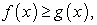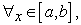then the definite integral of f from a to b is greater than or equal to the definite integral of g from a to b," which was proven by her teacher in class. The twelve students quoted similar attributes of various sizes of numbers, replacing the sizes of functions, to determine whether Statement 2 is correct. Take S10 as an example.

S10: When I see that the derivative of f is greater than that of g, I think of the size of numbers, e.g., 2 is greater than 1. Therefore, f(x) = 2x and g(x) = x, and 2x is greater than x.

I: Why is 2x greater than x?

S10: 2x is the double of x, so it is greater. For example, when x is equal to 1, 2 is greater than 1.

Their errors in intuitive strategies, called attribute substitution , occur when a more readily accessible attribute is substituted in a task for a less readily accessible attribute. For instance, similarity is a highly accessible attribute, because it is processed intuitively. S7 intuitively noticed the similarity between Statement 1 and mathematical theorems he knew. S10 noticed the similarity in the size of coefficient and function. Both of them replaced less accessible attributes with more accessible attributes. Similar to the students using property-based intuition, most of the students who noticed the similarity in the sizes of coefficient and function made relevance errors and neglected interval restrictions.

The interviewer subsequently asked students to draw graphs of algebraic function, in order to examine whether they could overcome intuitive relevance errors after visualizing mathematical statements. Results show that eight students overcame this error after drawing function graphs. Taking S10 as an example:

These are the graphs of f(x) and g(x), and f(x) is greater than g(x)...... No, that's not right. The graph below (referring to the graph below x axis) is something I didn't take notice of just now. From the graph, it can be seen that when x is negative, f(x) is beneath g(x), indicating that f(x) is less than g(x), so..... I am wrong. In fact, this statement is wrong. Although the derivative of f(x) (2) is greater than that of g(x) (1), it is not certain that within the interval including 0, e.g.,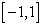or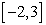, f(x) may be greater than g(x). How come I did not notice it just now? I noticed it only after drawing.

Therefore, graphical representation allowed S10 to understand the necessity of interval restrictions and overcome relevance errors. As for these students, the concreteness of visual images is an important factor for creating self-evidence and immediacy. A visual image not only organizes data at hand under a meaningful structure, but is also an important factor guiding the analytical development of a solution; visual representation serves as an important anticipatory device. The rest of the four students like S2 had difficulties in interpreting the dynamic relationship of the basic concepts of calculus. They relied on two types of interconnected schema (i.e., interval and property), but they were unable to integrate. Intuition exerts a coercive influence on the reasoning methods of individuals. An intuition subjectively generated by an individual is often a representation or interpretation that is absolute, while other representations or interpretations are excluded and unacceptable.

### 5. Discussion and Conclusion

The students in this study used three distinct types of intuitive reasoning to decide the truth value of mathematical statements. Each type of intuition provided students with a different starting point when approaching the tasks. With regard to logic-based intuition, we can conclude that for some students the equivalence between a statement and the converse is an intuition. With Fischbein , we can remark that the formal extrinsic type of conviction does not seem to have any effects on the intuitive intrinsic type of conviction, which can remain an obstacle. When using property-based intuition, the students based their decisions on vague ideas about properties in the task and always used graphical representations. Moreover, judging the truth value of mathematical statements and generating counterexamples by visualization is mediated by the intuition of the generality of the conclusions obtained by means of it. Similarity-based intuition was used when students identified a statement that was similar enough to the given statement to suggest the truth value of the given statement, but always used symbolic representations. Students' intuitive decision on the truth value of Statement 2 supports Buchbinder and Zaslavsky’s claim, is deeply rooted in the clues in the mathematical statement . From students' performance in this study, if the constructed intuitive representation accurately represents task structures, such related interpretations will have a positive effect on tasks, but if intuitive representations are distorted or deficient, they may lead to negative effects on reasoning.

Logical schema and bodies of mathematical knowledge are expected to develop and strengthen with age and /or instruction. Consequently, intuitive reasoning may lose its power in favor of other competing knowledge. However, in this study we could observe that some intuitive reasoning are resistant to age and experience evolution. These intuitive ways of reasoning may create difficulties in the acquisition and application of mathematical concepts.

In the students’ responses to the mathematical statements, we could observe that, in each intuitive response there seems to be an underlying logical schema, or a mental model intuitively accepted by the students, which interacts with the specific restrictions of the presented mathematical statement.

---The logical schemas of causality influenced the students reasoning strongly.

---The responses were influenced by intuitive rules that might have been the result of a more general tendency to extrapolate given information to new situations.

Mathematical statements like the ones posed in this study, which are known to elicit intuitive responses, should be presented to students in order to produce a cognitive conflict between their beliefs and the associated formal knowledge. The solution to this conflict, the analysis of the problem structure and the source of possible errors produced by intuition could help them overcome these difficulties and possibly generate more adequate intuitions.

### Acknowledgement

  Buchbinder, O. and Zaslavsky, O. “A framework for understanding the status of examples in establishing the validity of mathematical statements.” In Proceedings of the 33rd Conference of the International Group for the Psychology of Mathematics Education, PME, Vol. 2, 225-232, July, 2009.In article  Burton, L. Mathematicians as enquirers: Learning about learning mathematics, Kluwer, Boston, 2004.In article View Article  Cohen, L., Manion, L. and Morrison, K. Research methods in education (5th Ed). London, Routledge, 2000.In article View Article  de Villiers, M. Experimentation and proof in mathematics. In G. Hanna, H.N. Jahnke, & H. Pulte (Eds.). Explanation and proof in mathematics: Philosophical and educational perspectives. Springer, New York, 2010, 205-221.In article View Article  Durand-Guerrier, V., Boero, P., Douek, N., Epp, S., and Tanguay, D. Argumentation and proof in the mathematics classroom. In G. Hanna & M. de Villiers (Eds.), Proof and proving in mathematics education: The 19th ICMI study (pp. 349-367). Dordrecht, Netherlands, Springer. 2012.In article  Evans, J. “Intuition and reasoning: A dual-process perspective.” Psychological Inquiry, 21. 313-326.2010.In article  Fischbein, E. “Intuition and proof.” For the Learning of Mathematics, 3. 9-18. November. 1982.In article  Fischbein, E. Intuition in Science and Mathematics, Kluwer, Dordrecht, 1987.In article  Fischbein, E. The interaction between the formal, the algorithmic, and the intuitive components in a mathematical activity. In R. Biehler, R.W.,Scholz, R. Straser, & B. Winkelmann (Eds.). Didactics of mathematics as a scientific discipline. Kluwer, Dordrecht, 1994, 231-245.In article  Fischbein, E. “Intuitions and schemata in mathematical reasoning.” Educational Studies in Mathematics, 38. 11-50. March. 1999.In article  Glockner, A., and Witteman, C. “Beyond dual-process models: A categorization of processes underlying intuitive judgment and decision making.” Thinking and Reasoning, 16(1). 1-25. March. 2010.In article  Miles, B, M., and Huberman, A, M. “Drawing valid meaning from qualitative data: toward a shared craft.” Educational Researcher, 13(5). 20-30. May. 1984.In article  Tall, D. “The transition to formal thinking in mathematics.” Mathematics Education Research Journal, 20(2). 5-24. September. 2008.In article  Weber, K. “How syntactic reasoners can develop understanding, evaluate conjectures and generate counterexamples in advanced mathematics.” Journal of Mathematical Behavior, 28. 200-208. September. 2009.In article  Weber, K., and Alock, L. “Semantic and syntactic proof productions.” Educational Studies in Mathematics, 56. 209-234. July. 2004.In article